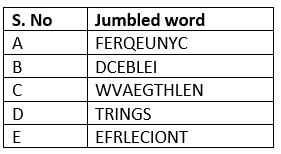# CBSE class 8 sound Worksheets

This page contains CBSE class 8 sound Worksheet  along with their answers. We also have notes for this chapter that can be accessed through links given below
Question 1
Fill in the blanks
1. The production of sound takes place due to ____________.
2. _________ is a fixed position where the oscillations produced.
3. Amplitude is measured in ___________.
4. The time period is measured in __________.
5. The number of oscillations takes place in one second is called __________.

Question 2
True and False
1. Frequency is measured in Hertz.
2. Shrill has a low frequency.
3. Male has a shrill voice.
4. The unit that is used to measure the loudness of sound is Hertz.
5. Wavelength is measured in Lambda.

Question 3
Unscramble the wordsQuestion 4
Name the following the words:
1. The time taken by any object to complete one oscillation.
2. The distance between two consecutive crests or troughs.
3. The frequency of sound that is above 20,000 Hz.
4. The frequency of sound that is below 20 Hz.
5. The maximum displacement covered by a particle from its mean position.
6. Two thin membranous structures that control the production of sound.
7. The sound is produced in this part of the human body.
8. Sound needs this to propagate.
9. The quality of the sound that depends on the source of sound.
10. An irritating sound that is unpleasant to ears.

Question 5
(i)What is decibel?
(ii)Write an equation that shows the relation between frequency and time period.
(iii)Give two examples of oscillatory motion.
(iv)What is gruff sound?
(v)Name three basic parts of human ear.

## Summary

This CBSE class 8 sound Worksheet is prepared keeping in mind the latest syllabus of CBSE . This has been designed in a way to improve the academic performance of the students. If you find mistakes , please do provide the feedback on the mail.

• Notes
• Assignments
• NCERT solutions